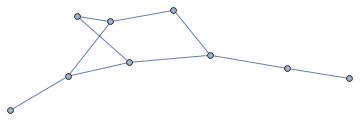# Using igraph from Mathematica

September 2015 update: If you are looking to use igraph from Mathematica, also take a look at the work-in-progress project IGraph/M. I now recommend using IGraph/M instead of IGraphR except when it does not yet have the functions you need.

This is a bit of promotion and a short tutorial for a tool I wrote that makes it easy to call the igraph graph library from Mathematica.

### Introdution

All software tools are buggy to some extent. This is definitely true for tools that are complex enough to be useful. Thus it is always important not to blindly trust results obtained with scientific software, but verify them.

In many cases verifying a result is much easier than obtaining it: for example, verifying eigenvectors and eigenvalues is as simple as substituting them back into $$Ax=\lambda x$$. In other cases the only practical option is to compute the result with alternative tools and check that it is the same. This is quite often true when doing graph/network related computations.

Mathematica has many functions for working with graphs and networks. It’s also been known to return wrong results sometimes (fixed in recent versions), like any other tool would. How can we make sure that the results it gives us are reliable? The best way is to compare with results from other software, but this was unfortunately a lot of work—until Mathematica 9 introduced RLink!

### IGraphR

igraph is one of the best open source graph manipulation and analysis packages, and it has a nice R interface, so we can call it through Mathematica’s RLink. I wrote a small package, IGraphR, to make this task much easier and much more convenient: it makes it possible to pass Mathematica graphs to igraph functions without modification, or receive graphs back as the result.

For example, creating an Erdős-Rényi random graph ($$G(n,m)$$ model) is as simple as

In[]:= IGraph["erdos.renyi.game"][10, 10, "gnm"]Find the edge-betweenness of all edges in the result:

In[]:= IGraph["edge.betweenness"][%]

Out[]= {8., 8., 7., 3.5, 5.5, 5.5, 9.5, 7., 14., 13.}


We can compare this result with the output of Mathematica’s builtin EdgeBetweennessCentrality, and discover that Mathematica gives twice the values igraph does. It turns out that for undirected graphs igraph’s definition of edge betweenness is the commonly used one. IGraphR is already proving useful!

In[]:= EdgeBetweennessCentrality[%%]

Out[]= {16., 16., 14., 7., 11., 11., 19., 14., 28., 26.}


### Setting up IGraphR

RLink comes with its own private installation of R. On systems other than Windows it is not possible to add the igraph package to this private R version, so we need to use an external R installation.

1. Start by downloading and installing R. Then start up R and evaluate install.packages('igraph') to install igraph, then close R.

2. Now we need to get Mathematica/RLink to use this external R installation.
Update: A complete guide on how to use an external R installation.

First load RLink using Needs["RLink"]. (If you’ve never used RLink before then evaluate RLinkResourcesInstall[] to automatically perform the initial setup. This will take a while and is only necessary the very first time RLink is used.) Then use the "RHomeLocation" option of InstallR to point it to the location of the external R installation. On OS X it is also necessary to specify the path to the libraries used by R:

SetEnvironment["DYLD_LIBRARY_PATH" -> "/Library/Frameworks/R.Framework/Resources/lib"];

InstallR["RHomeLocation" -> "/Library/Frameworks/R.Framework/Resources"];


When using Mathematica 10.0.1 (but not 10.0.0), specify the "RVersion" option as well, for example:

InstallR["RHomeLocation" -> "/Library/Frameworks/R.Framework/Resources", "RVersion" -> 3];

3. Install IGraphR: place the file IGRaphR.m in the directory opened by SystemOpen@FileNameJoin[{\$UserBaseDirectory, "Applications"}]

4. Evaluate Needs["IGraphR"] to load IGraphR. It’s ready to use!

### How to use

Simply wrap the name of an arbitrary R function with IGraph, and call it normally using Mathematica. For example, IGraph["vcount"][Graph[{1 <-> 2}]] will return the vertex count of the argument and IGraph["plot"][Graph[{"a" <-> "b"}]] will plot it using igraph. IGraphR ensures that any Mathematica graphs passed as arguments will be correctly converted to igraph graphs on the R side. If the return value is a single igraph graph, it will be converted back to a Mathematica graph.

While arbitrary R functions can be used, one would usually want to use this with one of the functions provided by the igraph library. They are listed on this page.

Currently IGraphR supports both directed and undirected graphs, as well as edge weights. Vertex names are preserved.

### Limitations

Mixed graphs, containing both directed and undirected edges, are not supported.

IGraphR is a simple extension of RLink, adding support for translating igraph graphs. Other than igraph graphs, it only supports data structures that RLink knows about. There are some igraph functions (such as V and E) which return other special data structures. These will either not transfer at all or will not transfer properly.